0
4641

# Venn Diagram Questions for SSC CHSL PDF:

SSC CHSL Venn Diagram Questions download PDF based on previous year question paper of SSC CHSL exam. 25 Very important Venn Diagram questions for SSC CHSL Exam.

SSC CHSL Study Material (FREE Tests)Question 1: Which of the following diagrams represents the relationship among Sun, Moon and Star?

a)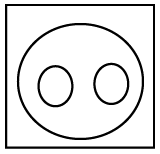b)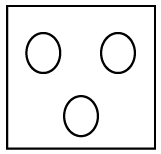c)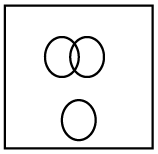d)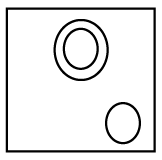Question 2: Which diagram correctly represents the relationship between Human beings, Teachers, Graduates? DIAGRAM

a)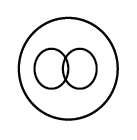b)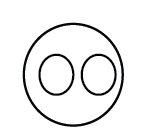c)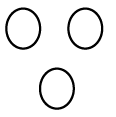d)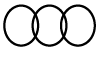Question 3: Which one of the following diagrams represents the correct relationship among Poison, Bio-products and Food ?

a)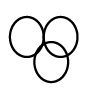b)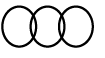c)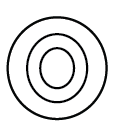d)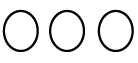Question 4: Which one of the following diagrams best depicts the relationship among pen,
pencils, stationery ?

a)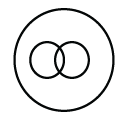b)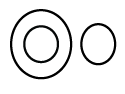c)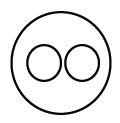d)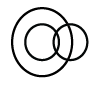Question 5: Indicate which figure will best represent the relationship amongst the three :
Legumes Seeds, Peas, Kidney Beans

a)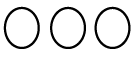b)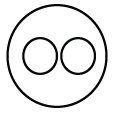c)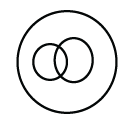d)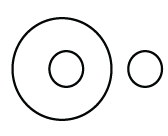Question 6: Which one of the following diagrams best depicts the relationship among
Black eyed people, Brown haired people, Indian

a)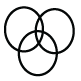b)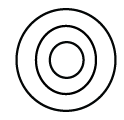c)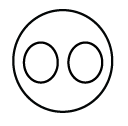d)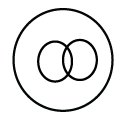Question 7: Identify the diagram that best represents the relationship among the given classes. Liquid, Pizza, Milk

a)

b)

c)

d)

Question 8: Identify the diagram that best represents the relationship among the given classes.Printer, Scanner, Input Device, Output Device, Computer.

a)

b)

c)

d)

Question 9: Identify the diagram that best represents the relationship among the given classes.
Complex number, Integer, Natural number

a)

b)

c)

d)

Question 10: Identify the diagram that best represents the relationship among the given classes. Music Instrument, Piano, Guitar

a)

b)

c)

d)Question 11: Identify the diagram that best represents the relationship among the given classes. Staff, Manager, Worker

a)

b)

c)

d)

Question 12: Identify the diagram that best represents the relationship among the given classes. Green, Mango, Fruits

a)

b)

c)

d)

Question 13: Identify the diagram that best represents the relationship among the given classes. Bull, Animal, Carnivorous

a)

b)

c)

d)

Question 14: Identify the diagram that best represents the relationship among the given classes. Animal, Leopard, Lion

a)

b)

c)

d)

Question 15: Identify the diagram that best represents the relationship among the given classes. Country, State, City

a)

b)

c)

d)

Question 16: Identify the diagram that best represents the relationship among the given classes. Professionals, Chartered Accountant, Female

a)

b)

c)

d)

Question 17: Identify the diagram that best represents the relationship among the given classes. Pet animals, Dog, Cat

a)

b)

c)

d)

Question 18: Identify the diagram that best represents the relationship among classes given below
Tennis fans, Cricket players, Students

a)

b)

c)

d)

Question 19: Identify the diagram that best represents the relationship among classes given below Males, Fathers, Engineers

a)

b)

c)

d)

Question 20: Identify the diagram that best represents the relationship among the given classes. Cricketer, Male, Actor

a)

b)

c)

d)Question 21: Identify the diagram that best represents the relationship among the given classes. Cap, Pencil, Apple

a)

b)

c)

d)

Question 22: Identify the diagram that best represents the relationship among the given classes. Four wheelers, Jeep, Car

a)

b)

c)

d)

Question 23: Identify the diagram that best represents the relationship among the given classes. Reptiles, Lizard, Lion

a)

b)

c)

d)

Question 24: Identify the diagram that best represents the relationship among the given classes. Biology, Science, Subject

a)

b)

c)

d)

Question 25: Choose the option which best captures the relationship between the following things.
1. Income Tax
2. Goods and Services Tax
3. Service Tax
4. Sales Tax
5. Import Duties

a)

b)

c)

d)Sun is a star, while moon is a planet.

=> The above relationship is depicted by :Ans – (D)

Both teachers and graduates are part part of human beings.

Also, some of the graduates can be teachers also and some may be not.

=> The above relation is correctly depicted by :Ans – (A)

Bio products can include food because this term is also related to agriculture. But all bio products are not food because it also includes making of fossil fuels, papers etc. We can also make different type of poison by using various bio products.

So, some bio products can be poison and some can be food.

Ans – (B)

Both pens and pencils are part of stationary, and also pens are different from pencils.

Thus, the venn diagram that best describe above relationship is :Ans – (C)

Both peas and kidney beans are part of legumes. Also peas and kidney beans are different.

Thus, the venn diagram the best describes their relation is :Ans – (B)

India can consist both black eyed people and brown haired people.

Similarly, A black eyed person can have brown hair and belong to India, and also a brown haired person can have black eyes and belong to India.

Thus, the venn diagram that best depicts above relationship is :Ans – (A)

Milk is in liquid form, thus all milk is liquid, and pizza is neither related to milk nor liquid. Thus, the last diagram best represents the relationship among the given classes.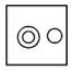=> Ans – (D)

Scanner is an input device and printer is an output device. Together, all these form a computer. Hence, option C best describes these relationships.

All natural numbers are integers and all integers are complex numbers.

Thus, the third figure represents above relationship.

=> Ans – (C)

Piano and Guitar are both different types of music instruments, thus the second figure best describe their relation.

=> Ans – (B)

All workers are staff members, and some workers are also managers, hence the first figure best describes above relationship.

=> Ans – (A)

All mango are fruits, whereas some mango are green and some fruits are also green.

Thus, the second figure best describe above relationship.

=> Ans – (B)

All bulls are animals, but bulls are not carnivorous.

Also, some carnivorous are animals, thus the last option is the best representation for above relationship.

=> Ans – (D)

Both leopard and lion are animals but are completely different from each other.

Thus, the third figure best describes above relationship.

=> Ans – (C)

All cities are part of a state and all states are part of a country.

Thus, the last figure perfectly describes the above relationship.

=> Ans – (D)

Both professionals and Chartered Accountants can be female, and all chartered accountants are professionals, hence the second diagram best describes above relationship.

=> Ans – (B)

All dogs are pet animals, similarly all cats are pet animals, but dogs are different from cats.

Thus, the first figure best represents above relationship.

=> Ans – (A)

A student can be both a tennis fan and a cricket player. Similarly, a tennis fan can be both a student and a cricket player.

Thus, all of the three are interrelated and first option best describes above relationship.

=> Ans – (A)

All fathers are definitely males, and the engineers can be either males or fathers.

Thus, the third figure best represents the above relationship.

=> Ans – (C)

A male can be a cricketer of an actor, but an actor cannot be a cricketer.

Thus, the venn diagram that best describes above relationship is :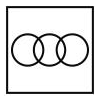=> Ans – (C)

Cap, pencil and apple are completely different from each other, hence do not intersect one another.

Thus, the venn diagram that best describes above relationship is :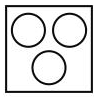=> Ans – (C)

Both jeep and car are different from each other, but comes under the category of four wheelers, hence the venn diagram that best describes above relation is :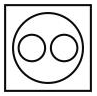=> Ans – (D)

All lizards comes under the category of lizards, but lions are mammals.

Thus, the venn diagram that best describes above relationship is :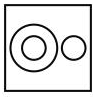=> Ans – (C)

Biology is a branch of science and science is a subject.

Thus, the venn diagram that best describes above relationship is :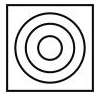=> Ans – (C)